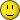6 Posts

## Subject: Mystical mapsDoes 'explore at distance of at most 3' mean that the the new explored tile is at distance 3 (an empty hex next to the board), or is it enough that the hex where one would ordinarily have the exploring hero standing is at distance 3?
• [+] Dice rolls
I don't understand the question.
• [+] Dice rolls
me neither. We need pictures• [+] Dice rolls
So you're asking whether normal explore would be "distance 0" or "distance 1"?
• [+] Dice rolls
Yes. I'm asking if regular explore is distance 0 or distance 1?

For mystical maps, are we counting distance from our hero to the hex at the edge of the current board (the existing hex next to which the new tile is supposed to get added), or are we counting to 'that plus 1'?
• [+] Dice rolls
I reckon the icon provides the answer. Normal explore is "distance 1".
• [+] Dice rolls